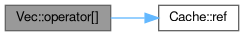Rcpp Version 1.0.7
Vec Class Reference

`#include <convolve10_cpp.h>`

Collaboration diagram for Vec:[legend]

## Public Types

typedef double & proxy

typedef double & proxy

## Public Member Functions

Vec (double *data_)

proxy operator[] (int i)

proxy operator[] (int i) const

Vec (double *data_)

double & operator[] (int i)

Vec (double *data_)

proxy operator[] (int i)

proxy operator[] (int i) const

Cache cache

double * data

## Detailed Description

Definition at line 16 of file convolve10_cpp.h.

## ◆ proxy [1/2]

 typedef double& Vec::proxy

Definition at line 18 of file convolve10_cpp.h.

## ◆ proxy [2/2]

 typedef double& Vec::proxy

Definition at line 31 of file convolve9_cpp.cpp.

## ◆ Vec() [1/3]

 Vec::Vec ( double * data_ )
inline

Definition at line 20 of file convolve10_cpp.h.

## ◆ Vec() [2/3]

 Vec::Vec ( double * data_ )
inline

Definition at line 16 of file convolve8_cpp.cpp.

## ◆ Vec() [3/3]

 Vec::Vec ( double * data_ )
inline

Definition at line 33 of file convolve9_cpp.cpp.

## ◆ operator[]() [1/5]

 double& Vec::operator[] ( int i )
inline

Definition at line 17 of file convolve8_cpp.cpp.

References data.

## ◆ operator[]() [2/5]

 proxy Vec::operator[] ( int i )
inline

Definition at line 21 of file convolve10_cpp.h.

References cache, and Cache::ref().

Here is the call graph for this function:## ◆ operator[]() [3/5]

 proxy Vec::operator[] ( int i )
inline

Definition at line 34 of file convolve9_cpp.cpp.

References cache, and Cache::ref().

Here is the call graph for this function:## ◆ operator[]() [4/5]

 proxy Vec::operator[] ( int i ) const
inline

Definition at line 22 of file convolve10_cpp.h.

References cache, and Cache::ref().

Here is the call graph for this function:## ◆ operator[]() [5/5]

 proxy Vec::operator[] ( int i ) const
inline

Definition at line 35 of file convolve9_cpp.cpp.

References cache, and Cache::ref().

Here is the call graph for this function:## ◆ cache

 Cache Vec::cache
private

Definition at line 25 of file convolve10_cpp.h.

Referenced by operator[]().

## ◆ data

 double* Vec::data
private

Definition at line 20 of file convolve8_cpp.cpp.

Referenced by operator[]().

The documentation for this class was generated from the following files: